Practice the problems of Math in Focus Grade 2 Workbook Answer Key Chapter 11 Money to score better marks in the exam.

Math Journal

Shawn made some mistakes in his homework. Help him correct the mistakes.

Example
Shawn’s mistake: 35¢ = $3.50 Correct answer: 35¢ =$0.35

Question 1.
Shawn’s mistake: One dollar and sixty cents = $1.06 Correct answer: _______ Answer: Correct answer is one dollar 06 cents. Question 2. Shawn’s mistake: 450¢ =$450
Correct answer is 450 cents = 450 cents.

Question 3.
Shawn’s mistake: $6 is$5 less than $10. Correct answer: ____________ Answer: Correct answer is$6 and $5 is less than$10.

Question 4.
Shawn’s mistake: $90 is$10 more than $100. Correct answer: _____________ Answer: Correct answer is$90 and $10 is more than$100.

Challenging Practice

Draw the amount of money using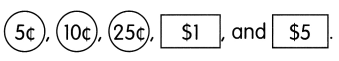Example
$5.70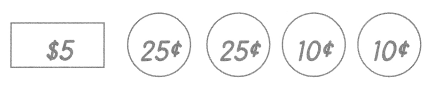Question 1.$4.60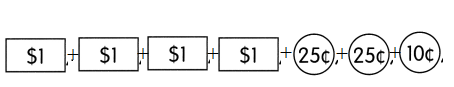$4.60 =$1 ++$1+$1+$1+ 25 cents + 25 cents + 10 cents. Question 2.$9.40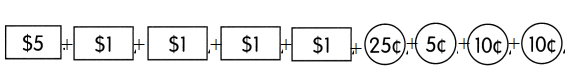$9.40 =$5+$1 +$1+$1+$1+ 25 cents + 5 cents + 10 cents + 10 cents.

Problem Solving

Find different combinations of coins and bills that can make each amount.

Example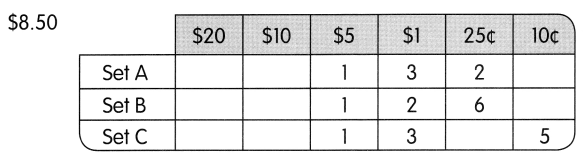Question 1.
$60.30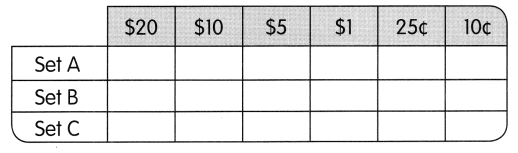Answer: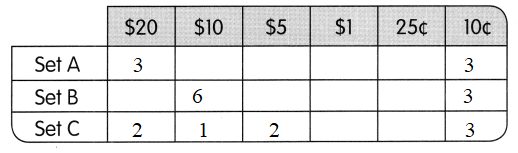Question 2.$25.00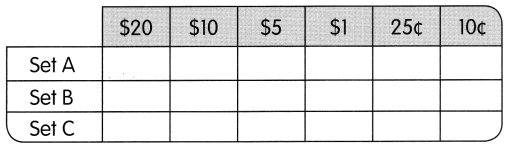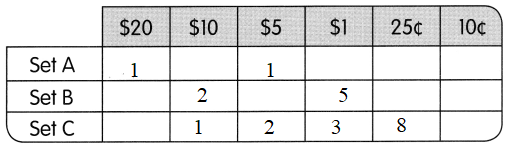Which amount is the greatest? _______
Answer: $60.30 is the greatest than$25.00

Chapter Review/Test

Vocabulary

Fill in the blanks with words or symbols from the box.

Question 1.
A _____ separates the dollars from the cents.
A decimal point separates the dollar from the cents.

Question 2.
100¢ makes _______ 1.
100 cents makes 1 dollar.

Question 3.
$.75 can also be written as 75 _____ Answer:$.75 cannot written has 75.

Concepts and Skills

Identify the value of each bill or coin.

Question 4.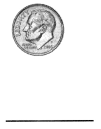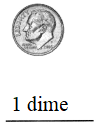1 dime

Question 5.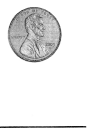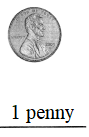1 penny

Question 6.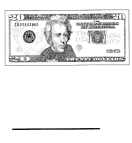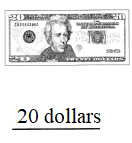20 dollars

Question 7.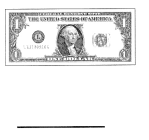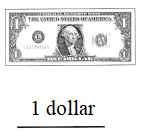1 dollar

Write the amount of money in words and numbers.

Question 8.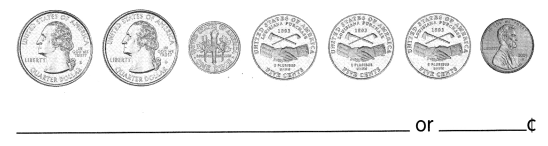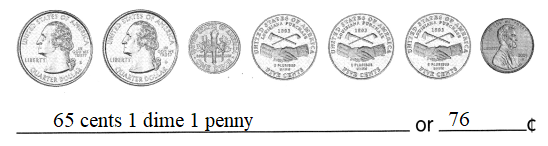Quarter dollar + quarter dollar + 1 dime + 5 cents + 5 cents + 5 cents + 1 penny
Quarter dollar = 25 cents
65 cents 1 dime 1 penny.
And
1 dime = 10cents
1 penny = 1cent
so
65 cents + 10cent + 1 cent =76 cent

Question 9.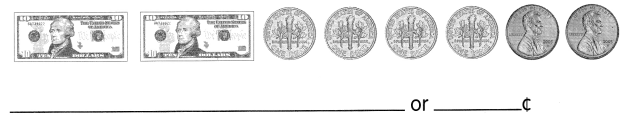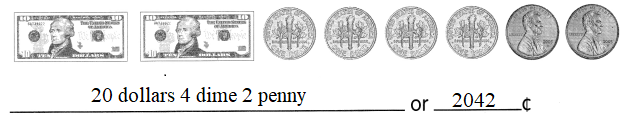10 dollars + 10 dollars + 1 dime + 1 dime + 1 dime + 1 dime + 1 penny + 1 penny
= 20 dollars 4 dime 2 penny
And
20 dollars = 2000cents
4 dime = 40cents
2penny = 2cents
so
2000cents + 40cents + 2cents = 2042 cent

Write the amount in dollars or cents.

Question 10.
50¢ = $_______ Answer: 50 cents = half dollar Question 11. 125¢ =$ _______
125cents = 1.25 dollars

Question 12.
$16 = _______¢ Answer:$16 = 1600 cents

Question 13.
$7.02 = ____ ¢ Answer: 702 cents Compare.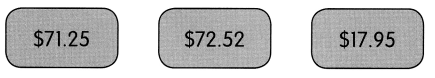Question 14. Which is the least amount? _____ Answer: 17.95 is the least amount. Question 15. Which is the greatest amount? ____ Answer:$72.52 is the greatest amount.

Problem Solving

Jeffrey has two $1 bills. He buys an ice cream for 70¢. How much does he have left? He has ____ left. Answer: Jeffrey has two$1 bills = $2 = 200 cent He buys an ice cream = 70 cents He have left = 200 cents – 70 cents = 130 cents. Question 17. Anita has$150. She spends $53 on books and$35 on a pair of shoes. How much does she have left?
She has $____ left. Answer: Anita has$150
She spends on books = $53 She spends on a pair of shoes =$35